## Limit Formula Result to Maximum or Minimum Value

This post will guide you how to limit formula result to a maximum or minimum value in excel. For example, if you want to limit the results of a formula to a maximum value of 200, how to this this in excel. How do I return a formula result only within a specified minimum or maximum values in excel. Do we have any ways to limit the results of a calculation to a maximum value.

You can use the MIN function or Max function to create a formula to limit a number within a minimum or maximum value. Just do the following steps:

## 1. Limit Formula Result to Maximum Value

If you want to limit a formula result to a maximum value 200, you can use the MIN function to create a formula, just like this:

``=MIN(200,SUM(B1:B3))``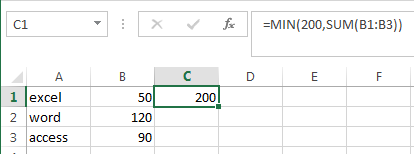If the result of SUM function is greater than 200, then the MIN function will return value 200. Otherwise, return the result of SUM function.

## 2. Limit Formula Result to Minimum value

If you want to limit a formula result (for example, the sum function) to a minimum value, then you can use the MAX function in combination with the SUM function or other function to create a new formula. Just like this:

``=MAX(200,SUM(B1:B3))``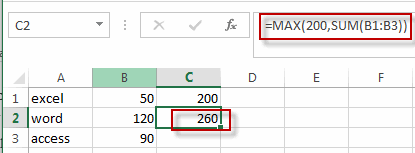If the result returned by the SUM function is smaller than 200, then returns value 200, otherwise, it will return the result returned by the SUM function.

## 3. Video: Limit Formula Result to Maximum or Minimum Value in Excel

This video will demonstrate how to limit the result of a formula to a specific minimum or maximum value using the MIN Function or MAX Function in Excel.

## 4. Related Functions

• Excel SUM function
The Excel SUM function will adds all numbers in a range of cells and returns the sum of these values. You can add individual values, cell references or ranges in excel.The syntax of the SUM function is as below:= SUM(number1,[number2],…)…
• Excel MIN function
The Excel MIN function returns the smallest numeric value from the numbers that you provided. Or returns the smallest value in the array.The MIN function is a build-in function in Microsoft Excel and it is categorized as a Statistical Function.The syntax of the MIN function is as below:= MIN(num1,[num2,…numn])….
• Excel MAX function
The Excel MAX function returns the largest numeric value from the numbers that you provided. Or returns the largest value in the array.= MAX(num1,[num2,…numn])…

## Make a Cumulative Chart

This post will guide you how to make a cumulative sum chart in Excel. How do I create a cumulative sum chart in Excel 2013/2016. How to add a cumulative total to a line chart in Excel.

## 1. Create a Cumulative Chart

Assuming that you have a list of data in range B1:C5 that contain sales data. And you want to create a cumulative sum chart for these data. How to achieve it. You just need to do the following steps:

Step1: select one blank cell next to the Sales column, and type the name of column D header as “TOTAL”.

Step2: type the following formula based on the SUM function in the Cell D2. And press Enter key in your keyboard, and then drag the AutoFill Handle from Cell D2 to D5.

``=SUM(C\$2:C2)``

Step3: select all data, and then go to INSERT tab, click Insert Column Chart command under Charts group. And select one 2-D Clustered Column. And one Column Chart has been created based on the selected data.

Step4: click Total Data series in the chart, and right click on it and select Change Series Chart Type from the popup menu list. The Change Chart Type dialog will open.

Step5: change the Chart type for TOTAL data series to Line, click OK button.

Step6: select the line data series, and right click on it. And select Add Data Labels, then select Add Data Labels sub menu.

Step7: Let’s see the result.

## 2. Video: Make a Cumulative Chart in Excel

This video will guide you step-by-step on how to make a cumulative sum chart in Excel to visualize the running total of data.

## 3. Related Functions

• Excel SUM function
The Excel SUM function will adds all numbers in a range of cells and returns the sum of these values. You can add individual values, cell references or ranges in excel.The syntax of the SUM function is as below:= SUM(number1,[number2],…)…

## How to Count Only Unique Values Excluding Duplicates in Excel

We enter a list of numbers or products and there are some duplicates in the list, if we want to just do count for the unique values and exclude the duplicates, how can we do? Now you can follow the below steps to solve this question by formula quickly.

Prepare a list of products and there are some duplicates among the list. See example below:

And we want to count the total product type in one year, how can we do count?

If we just use sum formula to do count, then duplicates will be included, so we need another formula to do count excludes the duplicates. See steps below.

## 1. Count Unique Values Excluding Duplicates by Formula in Excel

Step 1: In E2 which is saved the total product type number, enter the formula:

``=SUMPRODUCT(1/COUNTIF(B1:B11,B1:B11))``

Where B1:B11 is the range you want to count the unique values.

Step 2: Click Enter and get the result in E2. We can check the result is 6, and the duplicates are not included.

In above sample, we do count for unique values and get the result 6, because we have six products A\B\C\D\E\F, and if we want to only count the unique values exclude all duplicates like product A\B\C (they appeared more than one season) how can we do count? See below steps.

## 2. Count Unique Values Excluding All Duplicates by Formula in Excel

Step 1: In E2 which is saved the total product type number, enter the formula:

``=SUM(IF(FREQUENCY(MATCH(B1:B11,B1:B11,0),ROW(B1:B11)-ROW(B1)+1)=1,1))``

Where B1:B11 is the range you want to count the unique values.

Step2: Click Enter and get the result in E2. We can check the result is 3 (Product D\E\F are unique values and only appeared in one season).

## 3. Video: Count Only Unique Values Excluding Duplicates

If you want to learn how to count only unique values excluding duplicates in Excel, this video will show you a simple and effective formula that you can use in any situation.

## 4. Related Functions

• Excel SUMPRODUCT function
The Excel SUMPRODUCT function multiplies corresponding components in the given one or more arrays or ranges, and returns the sum of those products.The syntax of the SUMPRODUCT function is as below:= SUMPRODUCT (array1,[array2],…)…
• Excel COUNTIF function
The Excel COUNTIF function will count the number of cells in a range that meet a given criteria. This function can be used to count the different kinds of cells with number, date, text values, blank, non-blanks, or containing specific characters.etc.= COUNTIF (range, criteria)…
• Excel ROW function
The Excel ROW function returns the row number of a cell reference.The ROW function is a build-in function in Microsoft Excel and it is categorized as a Lookup and Reference Function.The syntax of the ROW function is as below:= ROW ([reference])….
• Excel MATCH function
The Excel MATCH function search a value in an array and returns the position of that item.The syntax of the MATCH function is as below:= MATCH  (lookup_value, lookup_array, [match_type])…
• Excel IF function
The Excel IF function perform a logical test to return one value if the condition is TRUE and return another value if the condition is FALSE. The IF function is a build-in function in Microsoft Excel and it is categorized as a Logical Function.The syntax of the IF function is as below:= IF (condition, [true_value], [false_value])….
• Excel SUM function
The Excel SUM function will adds all numbers in a range of cells and returns the sum of these values. You can add individual values, cell references or ranges in excel.The syntax of the SUM function is as below:= SUM(number1,[number2],…)…

## How to Count Cells that do not Contain Errors in Excel

This post will guide you how to count the number of cells that do not contain errors within a range of cells using a formula in Excel 2013/2016 or Excel office 365. How do I count the number of cells without any errors using a simple formula in Excel.

## 1. Count Number of Cells that do Not Contain Errors using Formula

You can use the SUMPRODUCT function in combining with NOT and ISERROR functions to count cells in a specified range of cells that do not contain errors

The below is a generic formula to count the number of cells that do not contain errors:

``=SUMPRODUCT(--NOT(ISERR(range)))``

Supposing that you wish to count the number of cells in range B1:B6 that do not contain errors, and you can use the following formula:

``=SUMPRODUCT(--NOT(ISERR(B1:B6)))``

LET’S SEE THAT HOW THIS FORMULA WORKS:

= NOT(ISERR(B1:B6))

The NOT and ISERROR functions can be used to identify the cells that do not contain an error. And it returns an array result with TRUE or FALSE values. The TRUE values indicate cells without errors, and FALSE values indicate cells with error values.

The array result is like this:

``{TRUE;TRUE;TRUE;FALSE;TRUE;FALSE}``

= –NOT(ISERR(B1:B6))

Then you still need to convert the above array result to their numeric values, 1 and 0, so that the SUMPRODUCT function can calculate the sum of products of corresponding numbers. And you can do this with a double negative operator. And the final array result is like this:

``{1;1;1;0;1;0}``

The SUMPRODUCT function sums the items in the above array and return the final result.

You can also use the SUM function to count the cell that do not contain errors in range B1:B6. And the generic formula is similar with the above formula. It need to be entered as an array formula through pressing `CTRL +SHIFT + Enter` to convert it as array formula. The formula looks like this:

``{=SUM(--NOT(ISERROR(B1:B6)))}``

## 2. Count Cells that do not Contain Errors using User Defined Function with VBA Code

If you want to count the number of cells that do not contain errors in a range, you can write a user defined function with VBA code. Here are the steps to create and run the function:

Step1: Press Alt + F11 keys to open the Microsoft Visual Basic for Applications window.

Step2: Click Insert > Module, and paste the following code in the Module Window.

```Function CountNonErrors_ExcelHow(rng As Range) As Long
Dim cell As Range
Dim count As Long
count = 0
For Each cell In rng
If Not IsError(cell.Value) Then
count = count + 1
End If
Next cell
CountNonErrors_ExcelHow = count
End Function```

Step3: Save and close the window.

Step4: In a blank cell, enter the formula:

``=CountNonErrors_ExcelHow(B1:B6)``

Where B1:B6 is the range you want to count.

Step5: Press Enter to get the result. This function will return the number of cells that do not contain errors in the specified range.

## 3. Video: Count Cells that do not Contain Errors in Excel

This video will show you how to count cells that do not contain errors in Excel using a simple formula and a VBA code.

## 4. Related Functions

• Excel SUMPRODUCT function
The Excel SUMPRODUCT function multiplies corresponding components in the given one or more arrays or ranges, and returns the sum of those products. The syntax of the SUMPRODUCT function is as below:= SUMPRODUCT (array1,[array2],…)…
• Excel ISERROR function
The Excel ISERROR function used to check for any error type that excel generates and it returns TRUE for any error type, and the ISERR function also can be checked for error values except #N/A error, it returns TRUE while the error is #N/A. The syntax of the ISERROR function is as below:= ISERROR (value)….
• Excel SUM function
The Excel SUM function will adds all numbers in a range of cells and returns the sum of these values. You can add individual values, cell references or ranges in excel.The syntax of the SUM function is as below:= SUM(number1,[number2],…)…
• Excel NOT function
The Excel NOT function returns the opposite of a given logical or Boolean value. For example, if you supplied with the value TRUE, the NOT function will return FALSE; If you supplied with the value FALSE, and the NOT function will TRUE. The syntax of the NOT function is as below:=NOT(logical)…

## How to Count Numbers with Leading Zeros

This post will guide you on how to count numbers with leading zeros in Excel 2013/2016/2019/365. When working with numbers that have leading zeros, it can be challenging to count the number of zeros that appear before the actual value. Fortunately, there are two main methods for counting leading zeros in Excel: using a formula or using VBA code.

In this post, we’ll walk through the steps for each method, so you can choose the one that works best for your needs.

## 1. Count Numbers with Leading Zeros using Formulas

Suppost you have a list of product ID in your worksheet, and the product ID contains the different number of leading zeros in the front of string. And if you use the COUNTIF function to count numbers with leading zeros, but it will remove those leading zeros, it means that 1289 and 001289 are the same strings.

So how to preserve the leading zeros while counting numbers. And you can use another Excel function named as SUMPRODUCT.

For example, you want to count strings with leading zeros in the range A2:A6, you can write down the following formula based on the SUMPRODUCT function.

``=SUMPRODUCT(--(\$A\$2:\$A\$6=A2))``

You can enter this formula into Cell B2, then press Enter key. Then you can drag AutoFill Handle down to other cells to apply this formula.

You can also use another excel array formula to count strings with leading zeros as follows:

``=SUM(IF(\$A\$2:\$A\$10=A2,1,0))``

## 2. Count Numbers with Leading Zeros using User Defined Function with VBA Code

Step1: press ALT+F11 to open the VBA editor.

Step2: Click on Insert -> Module to create a new module.

Step3: Copy and paste the following code into the module:

```Function CountLeadingZeros_ExcelHow(cell As Range) As Long
Dim count As Long
Dim value As String
value = cell.value
count = 0
While Left(value, 1) = "0" And Len(value) > 1
count = count + 1
value = Mid(value, 2)
Wend
If Left(value, 1) = "0" Then count = count + 1
End Function```

The VBA code uses a While loop to count the number of leading zero characters in the specified cell. And it checks whether the leftmost character of the cell’s value is “0” and whether the length of the cell’s value is greater than 1.

If both conditions are true, it increments a count variable and removes the leftmost character from the cell’s value using the Mid function.

Step4: Save the module and go back to your Excel worksheet.

Step5: Type the following formula in a blank cell to count the number of leading zero characters in cell A2.

``=CountLeadingZeros_ExcelHow(A2)``

Step6: Press Enter to see the result.

## 3. Video: Count Numbers with Leading Zeros

This video explains how to count numbers with leading zeros in Excel using a formula or VBA code. And it provides step-by-step instructions for both methods and demonstrates how to use them in practice.

## 4. Related Functions

• Excel SUMPRODUCT function
The Excel SUMPRODUCT function multiplies corresponding components in the given one or more arrays or ranges, and returns the sum of those products.The syntax of the SUMPRODUCT function is as below:= SUMPRODUCT (array1,[array2],…)…
• Excel COUNTIF function
The Excel COUNTIF function will count the number of cells in a range that meet a given criteria. This function can be used to count the different kinds of cells with number, date, text values, blank, non-blanks, or containing specific characters.etc.= COUNTIF (range, criteria)…
• Excel IF function
The Excel IF function perform a logical test to return one value if the condition is TRUE and return another value if the condition is FALSE. The IF function is a build-in function in Microsoft Excel and it is categorized as a Logical Function.The syntax of the IF function is as below:= IF (condition, [true_value], [false_value])….
• Excel SUM function
The Excel SUM function will adds all numbers in a range of cells and returns the sum of these values. You can add individual values, cell references or ranges in excel.The syntax of the SUM function is as below:= SUM(number1,[number2],…)…

## 5. Related Posts

• How to insert leading zeros to number or text
Assuming that you want to insert leading zeros  to number in Cell A2, then you can try to use the TEXT function to create an excel formula. You can also use the CONCATENATE function to add the specific digit of leading zeros into each number…
• How to keep or remove leading zeros
If you want to keep the leading zeros as you type in a cell, you need to change the cell format to Text before you type the zip code number.You can remove or delete leading zeros in front of the cells with excel VBA macro…
• Count the number of words in a cell
If you want to count the number of words in a single cell, you can create an excel formula based on the IF function, the LEN function, the TRIM function and the SUBSTITUTE function. ..

## How to Sum in Vertical Range

If we want to add numbers based on some conditions in Excel worksheet, we can add criteria with the help of SUMIFS function, SUMIFS can filter data with multiple criteria effectively. In this article, we will introduce you the method about applying SUMIFS function to sum numbers with different vertical ranges.

We will introduce you the syntax, arguments of SUMIFS function, and let you know how this formula works to reach your goal. After reading the article, you may have a simple understanding of SUMIFS function and know how can we use this function to sum numbers with vertical range.

## 1. Sum in Vertical Range Example

Refer to the upper side table, it lists the amount for ‘Apple Pie’, ‘Hamburger’ and ‘Salad’ on weekdays (from ‘Monday’ to ‘Friday’) in two weeks. Start from column C, it lists the amount for the three kinds of food on each weekday for two weeks in vertical direction. In the bottom side table, it records the total amount for the three kinds of food in two weeks.

Now, we need to create a formula that can return the total amount for each food in two weeks based on different weekdays. This question can be seen as we need to sum data from a vertical range with specific criteria. For example, in this instance, in C9, we want to enter a formula that can return the total amount for ‘Apple Pie’ on ‘Monday’ in ‘two weeks’ (week1 and week2). Besides, when dragging the formula to other cells (in range reference C9 to G11), we need the formula can adjust cell reference and range reference automatically and return the correct result based on updated parameters properly.

In this instance, we need to pick up numbers with criteria from each vertical range, then sum them together. To fix this issue by formula, we can apply excel built-in function SUMIFS here.

## 2. Sum in Vertical Range Formula – SUMIFS FUNCTIONS

Step1: In C9, enter the formula

``=SUMIFS(C\$2:C\$7,\$B\$2:\$B\$7,\$B9)``

Step2: Press Enter after typing the formula.

We can see in cell C9 result 9 is returned, it is equal to 5 from C2 plus 4 from C4, the formula works correctly.

Step3: Drag the ‘Auto Fill’ handle to make range reference C9:G11 filled with the formula. Then we can see that results are automatically calculated and displayed in each cell correctly.

## 3. SUMIFS FUNCTION INTRODUCTION

SUMIFS function can be seen as SUM+IFS, it can handle multiple ‘criteria range’ and ‘criteria’ combinations.

Syntax:

``=SUMIFS(sum_range, criteria_range1, criteria1, [criteria_range2, criteria2], ...)``

[] part can be omitted.

For SUMIFS function, it supports wildcards like asterisk ‘*’ and question mark ‘?’, also support logical operators. If wildcards or logical operators are required, they should be enclosed into double quotes (““).

The usage of wildcards:

1. An asterisk (*) means one or more characters.
2. A question mark (?) means one character.
3. The position of asterisk or question mark means the character(s) position relative to the entered part.

The usage of logical operators:

1. “>” – greater than
2. “<” – less than
3. “<>” – not equal to

ALL ARGUMENTS

The formula is =SUMIFS(C\$2:C\$7,\$B\$2:\$B\$7,\$B9). Refer to above SUMIFS function introduction, we will split it to several parts.

### SUMIFS – SUM RANGE

C\$2:C\$7 is the ‘sum range’ in this case.

You can see we add “\$” before row index 2 and 7, that’s because when dragging the formula from C9 to C10 (formula is moved in vertical direction), cell reference will be moved in vertical direction by default, so we need add “\$” before row index to lock the range in vertical direction, make sure the sum range can only be adjusted in vertical, but in horizontal, it is absolutely fixed.

See screenshot below, if “\$” is omitted, formula is updated to =SUMIFS(C3:C8,\$B\$2:\$B\$7,\$B10) in C10, sum range is moved from range reference C2:C7 to C3:C8 improperly, the returned result is 9 incorrectly.

Select C\$2:C\$7 from formula in the formula bar, the press F9, values in this range are expanded in an array.

### SUMIFS – CRITERIA RANGE

\$B\$2:\$B\$7 is the criteria range. We have only one criteria range in this instance.

Criteria range contains three kinds of food, due to there are two weeks, so we list them twice.

You can see we add “\$” before column and row index both, that’s because range \$B\$2:\$B\$7 is an absolute range. No matter copy formula to which cell reference, the criteria range is still \$B\$2:\$B\$7 and will not be changed. So we add ‘\$’ to lock this range.

Select \$B\$2:\$B\$7 from formula in the formula bar, the press F9, values in this range are expanded in an array.

### SUMIFS – CRITERIA

\$B9 is the criteria. \$B9=”Apple Pie”.

As we want to calculate total amount for the provided food, so can set criteria as B9 (B9=”Apple Pie”), B10 (B10=”Hamburger”), and B11 (B11=”Salad”). As column B is fixed, so we add ‘\$’ before column B.

We can also enter food name directly, as SUMIFS function supports texts, but they should be enclosed into double quotes “”, so for criteria “Apple Pie”, we can directly enter “Apple Pie” in criteria argument.

Select \$B9 from formula in the formula bar, the press F9, criteria value is displayed.

## 4. Sum in Vertical Range – Formula Explantation

After explaining each argument in the formula, now we will show you how the formula works with these arguments.

After expanding values in each range reference or cell reference, in the formula bar, the formula is displayed as:

``=SUMIFS({5;10;5;4;12;4},{"Apple Pie";"Hamburger";"Salad";"Apple Pie";"Hamburger";"Salad"},"Apple Pie")``

There is one pair of criteria range and criteria.

{“Apple Pie”} – Criteria

If values from the criteria range are matched with the criteria “Apple Pie”, “True” will be recorded and saved, otherwise, “False” will be saved instead. So, after comparing, we can get a new array:

{True;False;False;True;False;False} – after comparing with criteria “Apple Pie”

For the following logical operation, “True” is coerced to ‘1’ and ‘False’ is coerced to ‘0’. So above array is converted to below array which consists of numbers “1” and “0”.

{1;0;0;1;0;0} – for criteria “Apple Pie”

Now, we have below two pairs of arrays:

{5;10;5;4;12;4} – sum range

{1;0;0;1;0;0} – for criteria “Apple Pie”

Multiply the two elements in the same position in the two arrays. Then we can get a new array.

{5;0;0;4;0;0}

Add all products in above array, we get 9 finally. Actually, in step#2, SUMIFS function returns 9 after calculation. For the other cells, the workflow is similar.

1. In this case, as we have only one pair of criteria range and criteria, we can also apply SUMIF function as well. The difference is SUMIF function only supports one group of criteria range and criteria, and its sum range is listed at the end.

Enter =SUMIF(\$B\$2:\$B\$7,\$B9,C\$2:C\$7), we can get the same result as applying SUMIFS.

## 5. Video: How to Sum in Vertical Range in Excel

In this video, you will learn how to use the SUMIF formula to sum values in a vertical range in Excel.

## 6. Related Functions

• Excel SUMIF Function
The Excel SUMIF function sum the numbers in the range of cells that meet a single criteria that you specify. The syntax of the SUMIF function is as below:=SUMIF (range, criteria, [sum_range])…
• Excel SUMIFS Function
The Excel SUMIFS function sum the numbers in the range of cells that meet a single or multiple criteria that you specify. The syntax of the SUMIFS function is as below:=SUMIFS (sum_range, criteria_range1, criteria1, [criteria_range2, criteria2], …)…
• Excel SUM function
The Excel SUM function will adds all numbers in a range of cells and returns the sum of these values. You can add individual values, cell references or ranges in excel.The syntax of the SUM function is as below:= SUM(number1,[number2],…)

## How to Sum the Smallest N Values in Excel

Sometimes we may want to sum the first smallest N numbers in a range in Excel. In this article, we will show you the method of “SUM the Smallest N Numbers” by a simple formula which consist of SUMPRODUCT and SMALL functions. SMALL can return smallest values based on criteria, SUMPRODUCT can sum up these smallest values.

Through a simple instance, we will introduce you the syntax, argument and the usage of SUMPRODUCT and SMALL functions. We will let you know how the formula works step by step clearly. After reading the article, you may know that in which situations we can choose SUMPRODUCT function to sum data.

## 1. Sum the Smallest N Values Using SUM Formula

In the ‘Value’ column, there are 10 values from ‘1’ to ‘10’ in improper order. Now we want to sum the smallest 4 values from this list, how can we get the correct result?

Actually, we can sort these numbers from smallest to largest firstly by ‘Sort A-Z’ in excel.

Step1: Click ‘Data’ tab, under ‘Sort & Filter’ section click ‘Sort Smallest to Largest’ icon.

Step2: Then you can find numbers are ordered properly.

Step3: Then you can enter SUM function in B2 and select the first four numbers ->cell reference A2:A5 in the list.

Step4: Then you can get the sum of the smallest four values.

## 2. Sum the Smallest N Values Using SUMPRODUCT Formula

We can also get sum conveniently and correctly by just enter a formula. As we want to sum the smallest four numbers from range A2:A11, we can find the smallest N number or numbers by SMALL function actually, and then use SUMPRODUCT function to sum array directly.

Step1: In B2, enter the formula

``=SUMPRODUCT(SMALL(A2:A11,{1,2,3,4}))``

Step2: Press Enter after typing the formula.

We can see that this time we also get correct result 10. The formula works correctly.

HOW THIS FORMULA WORKS

The formula is converted as:

=SUMPRODUCT(SMALL({1;3;5;6;9;10;7;8;4;2},{1,2,3,4}))

Now we will show you how the formula works with the two functions.

For SMALL({1;3;5;6;9;10;7;8;4;2},{1,2,3,4}), refer to k value {1,2,3,4}, we find out the smallest 4 values in the array {1;3;5;6;9;10;7;8;4;2}.

Select SMALL({1;3;5;6;9;10;7;8;4;2},{1,2,3,4}) in the formula bar, press F9. We can get the result {1,2,3,4}.

Obviously, the final result is 1+2+3+4=10. Select SUMPRODUCT({1,2,3,4}) in the formula bar, press F9, 10 is displayed in formula bar.

## 3. Sum the Smallest N Values in Excel with User Defined Function with VBA Code

You can create a user-defined function in Excel to sum the smallest N values in a range of cells. Here are the steps:

Step1: Press Alt + F11 to open the Visual Basic Editor.

Step2: Click Insert > Module to insert a new module.

Step3: Copy and paste the following code into the module. Save the module and close the Visual Basic Editor.

```Function SumSmallestN_Excelhow(rng As Range, n As Integer) As Double
Dim i As Integer
Dim j As Integer
Dim k As Integer
Dim temp As Double
Dim sum As Double

For i = 1 To n
For j = i To rng.Cells.count - 1
If rng.Cells(j).Value < rng.Cells(i).Value Then
temp = rng.Cells(i).Value
rng.Cells(i).Value = rng.Cells(j).Value
rng.Cells(j).Value = temp
End If
Next j
sum = sum + rng.Cells(i).Value
Next i

SumSmallestN_Excelhow = sum
End Function```

Step4: enter the formula into a blank cell:

``=SumSmallestN_Excelhow(A2:A11,4)``

Where A2:A11 is the range of cells you want to sum and 4 is the number of smallest values you want to sum.

Step5: Press Enter to calculate the sum of the smallest 4 values in the range.

## 4. Video: Sum the Smallest N Values in Excel

This video will demonstrate how to sum the smallest N values in Excel using a formula or  a User Defined Function with VBA code as the formula.

## 5. Related Functions

• Excel SUMPRODUCT function
The Excel SUMPRODUCT function multiplies corresponding components in the given one or more arrays or ranges, and returns the sum of those products.The syntax of the SUMPRODUCT function is as below:= SUMPRODUCT (array1,[array2],…)…
• Excel INDIRECT function
The Excel INDIRECT function returns the cell reference based on a text string, such as: type the text string “A2” in B1 cell, it just a text string, so you can use INDIRECT function to convert text string as cell reference….
• Excel ROW function
The Excel ROW function returns the row number of a cell reference.The ROW function is a build-in function in Microsoft Excel and it is categorized as a Lookup and Reference Function.The syntax of the ROW function is as below:= ROW ([reference])….
• Excel SMALL function
The Excel SMALL function returns the smallest numeric value from the numbers that you provided. Or returns the smallest value in the array.The syntax of the SMALL function is as below:=SMALL(array,nth) …
• Excel SUM function
The Excel SUM function will adds all numbers in a range of cells and returns the sum of these values. You can add individual values, cell references or ranges in excel.The syntax of the SUM function is as below:= SUM(number1,[number2],…)

## Count Non-Contiguous Range using COUNTIF

This post will guide you how to count a non-contiguous range with criteria in COUNTIF function in Excel 2013/2016 or Excel office 365. How do I build a formula for counting non-contiguous range in Excel using COUNTIF function or User Defined Function in Excel.

## 1. What is Non-Contiguous Range?

A non-contiguous range consists of tow of more separate ranges in your current worksheet. And this post will show you one formula that counting the non-contiguous range with criteria in Excel.

## 2. Generic Formula

If you want to count a non-contiguous range with criteria, and you can use the COUNTIF function in combination with the INDIRECT and the SUM function. The general formula is like this:

``=SUM(COUNTIF(INDIRECT({“range1″,”range2″,”range3”}),criteria))``

The syntax of Explanations is as below:

• SUM – the sum function can be used to add up values in the given cells.
• COUNTIF – COUNT the number of cells that match a certain condition.
• INDIRECT – return a valid cell reference from a given text string.

## 3. COUNT Non-Contiguous Range Using COUNTIF

Assuming you have tree ranges A1:A5, C1:C5, and E1:E5 in your worksheet and you wish to count the number of values that is greater than 80. And you can use the below formula:

``=SUM(COUNTIF(INDIRECT({"A1:A5","C1:C5","E1:E5"}),">80"))``

Let’s See That How This Formula Works:

The COUNTIF function can only count the number of cells in a range that match a given condition in Excel. And if you want to use the COUNTIF function with non-contiguous ranges, and you will get an error value. The best method is that you can use the INDIRECT function to get a valid cell reference from an array list that contain multiple text string.

The multiple ranges that returned by the INDIRECT function are passed into COUNTIF function. As the COUNTIF function receives an array list, it will return an array result also.

Finally, you can use the SUM function to add up all items in an array.

You can also use another solution to count Non-contiguous range using COUNTIF function. And using more that one COUNTIF function to count the numbers. The formula is like this:

``=COUNTIF(A1:A5,">80")+COUNTIF(C1:C5,">80")+COUNTIF(E1:E5,">80")``

You can also use the SUMPRODUCT function along with the COUNTIF function to count non-contiguous ranges in Excel. Just use the following formula:

``=SUMPRODUCT(COUNTIF(INDIRECT({"A1:A5","C1:C5","E1:E5"}),">80"))``

This formula will count the number of cells that meet your criteria in the non-contiguous ranges that you specify.

## 4. Count Non-Contiguous Range with VBA Code

You can count non-contiguous ranges in Excel with VBA code (user-defined function), just do the following steps:

Step1: Press the Alt and F11 keys at the same time to open the VBA editor.

Step2: In the VBA editor, select “Insert” from the menu bar and choose “Module” to create a new module.

Step3: Copy and paste the VBA code for the user-defined function into the module. Save the workbook with the VBA code.

```Function CountValuesAboveCriteria_excelHow(rngArray As Variant, criteria As Variant) As Long
Dim countArray() As Long
ReDim countArray(LBound(rngArray) To UBound(rngArray))

Dim i As Long
For i = LBound(rngArray) To UBound(rngArray)
countArray(i) = Application.CountIf(Range(rngArray(i)), criteria)
Next i

CountValuesAboveCriteria_excelHow = Application.Sum(countArray)
End Function```

Step4: Close the VBA editor and return to the worksheet where you want to use the function.

Step5: In any blank cell, enter the formula:

``= CountValuesAboveCriteria_excelHow(rngArray, criteria) ``

Where “rngArray” is an VBA array of range strings (e.g. {“A1:A5″,”C1:C5″,”E1:E5”}) and “criteria” is the criteria string (e.g. “>80“).

``=CountValuesAboveCriteria_excelHow({"A1:A5","C1:C5","E1:E5"},">80")``

Step6: Press Enter to see the result.

## 5. Video: Count Non-Contiguous Range in excel

This video will demonstrate both formula and VBA code methods for counting non-contiguous ranges in Excel.

## 6. Related Functions

• Excel SUM function
The Excel SUM function will adds all numbers in a range of cells and returns the sum of these values. You can add individual values, cell references or ranges in excel.The syntax of the SUM function is as below:= SUM(number1,[number2],…)…
• Excel COUNTIF function
The Excel COUNTIF function will count the number of cells in a range that meet a given criteria. This function can be used to count the different kinds of cells with number, date, text values, blank, non-blanks, or containing specific characters.etc.= COUNTIF (range, criteria)…
• Excel INDIRECT function
The Excel INDIRECT function returns the cell reference based on a text string, such as: type the text string “A2” in B1 cell, it just a text string, so you can use INDIRECT function to convert text string as cell reference….

## How to Calculate the Average Excluding the Smallest & Highest Numbers in Excel

This post will introduce you to three methods for calculating the average of a range of numbers in Microsoft Excel, excluding the smallest and largest values. The first and second method involves using the formula, while the third method utilizes VBA code.

## 1. Calculate the Average Excluding the Smallest and Highest Numbers Using Formula

Calculating the average for a batch of data is frequently used in our daily life. But for some cases like statistic the average score in a competition, or price analysis, we often calculate the average excluding the smallest and highest numbers in the range of data.

As we know we can use some formula like MAX or MIN to get the maximum value or minimum value in a range, so we can use formula combination to calculate the average excluding the MAX and MIN values. In this article, we will provide you some useful formulas to solve our problem.

We can use the total amount minus the smallest value and the highest value, then divide the total number-2, so for this case we can use the similar formula combination to calculate the average. Details see steps below.

Step1: In any blank cell, enter the following formula.

`` =(SUM(A2:A9)-MIN(A2:A9)-MAX(A2:A9))/(COUNT(A2:A9)-2)``

Step 2: Click Enter to get the result. Verify that average is calculated properly.

## 2. Calculate the Average Excluding the Smallest and Highest Numbers Using TRIMMEAN Function

Above formula combination is a little complex, we can use a special function TRIMMEAN to calculate the average for some conditions.

Step 1: In any blank cell, enter the formula

``=TRIMMEAN(A2:A9,2/COUNT(A2:A9))``

Step 2: Click Enter to get the result. Verify that average is calculated properly. It is the same with the value calculated by the formula combination.

## 3. Calculate the Average Excluding the Smallest and Highest Numbers with VBA Code

If we frequently use the function to calculate the average in daily work, we can create a user defined function by VBA, then we can use the function directly.

Step1: Click Developer tab->Visual Basic or Alt+F11 to load Microsoft Visual Basic for Applications window.

Step2: Click Insert->Module to insert a module. Then Module1 is created.

Step3: Enter below code in Module window.

```Function AverageExcludeMaxAndMin_excelhow(myrange) As Variant
With Application
AverageExcludeMaxAndMin_excelhow = (.Sum(myrange) - .Max(myrange) - .Min(myrange)) / (.Count(myrange) - 2)
End With
End Function```

In above code, we create the function as AverageExcludeMaxAndMin_excelhow. You can shorten the function name per your requirement.

Step4: In any blank cell, enter =Average, verify that user defined function AverageExcludeMaxAndMin_excelhow is loaded.

Step5: In any blank cell, enter the formula:

`` =AverageExcludeMaxAndMin_excelhow(A2:A9)``

Step6: Click Enter to get the result.

So after above steps, we can directly use this function to calculate the average in following work directly.

## 4. Video: Calculate the Average Excluding the Smallest and Highest Numbers

This video will demonstrate how to use a User Defined Function (UDF) and formula to calculate the average of a range of numbers in Excel, excluding the smallest and largest values.

## 5. Conclusion

These methods are useful when you want to calculate the average of a range of values but want to exclude any outliers that could skew the result. By following the steps outlined in this post, you can easily calculate the average of a range of values in Excel while excluding the smallest and largest numbers.

## 6. Related Functions

• Excel SUM function
The Excel SUM function will adds all numbers in a range of cells and returns the sum of these values. You can add individual values, cell references or ranges in excel.The syntax of the SUM function is as below:= SUM(number1,[number2],…)…
• Excel MIN function
The Excel MIN function returns the smallest numeric value from the numbers that you provided. Or returns the smallest value in the array.The MIN function is a build-in function in Microsoft Excel and it is categorized as a Statistical Function.The syntax of the MIN function is as below:= MIN(num1,[num2,…numn])….
• Excel MAX function
The Excel MAX function returns the largest numeric value from the numbers that you provided. Or returns the largest value in the array.= MAX(num1,[num2,…numn])…
• Excel COUNT function
The Excel COUNT function counts the number of cells that contain numbers, and counts numbers within the list of arguments. It returns a numeric value that indicate the number of cells that contain numbers in a range…

## Show Only Positive Values

This post will guide you on how to show only positive values in Excel. There are several ways to accomplish this, including formatting cells, using conditional formatting, and using VBA code.

We will discuss each method in detail and provide step-by-step instructions on how to implement them.

## 1. Video: Show Only Positive Values

In this video, we will explore various methods, such as formatting cells, using conditional formatting, and VBA code, to show only positive values in Excel.

## 2. Show Only Positive Numbers in a Range with Format Cells

To only display positive numbers in a range using the Format Cells feature in Microsoft Excel, follow these steps:

Step1: Select one range that you want to format.

Step2: Right-click and select “Format Cells” from the context menu.

Step3: In the Format Cells dialog box, select the “Number” tab. Select “Custom” from the Category list. In the “Type” field, enter the following format code: 0;[White0 , Click “OK” to apply the formatting.

Step3: only positive numbers in the selected range will be displayed.

## 3. Show Only Positive Numbers in a Range with Conditional Formatting

If you only want to highlight or format positive numbers in a range, you can also use the Conditional Formatting feature in Microsoft Excel, just do the following steps:

Step1: Select one range that want to format.

Step2: Click on the “Conditional Formatting” button in the “Home” tab of the ribbon. Select “Highlight Cell Rules” and then “Less Than” from the dropdown menu.

Step3: In the “Less Than” dialog box, enter “0” in the “Value” field. Choose “Custom Format…”, then set the font color as white.

Step3: Click “OK” to apply the formatting.

This will only show all cells in the selected range that contain a value greater than zero.

## 4. Show Only Positive Numbers in a Range with VBA Code

If you want to show positive numbers in a range using VBA code, you can use a loop to iterate through each cell in the range and check if the value is greater than zero. If the value is positive, you can keep it in the cell. Otherwise, you can set font color as white.

Just refer to the following steps:

Step1: Press Alt + F11 on your keyboard. This will open the Visual Basic Editor.

Step2: Go to the menu bar at the top of the Visual Basic Editor and click on Insert -> Module. This will create a new module in the project.

Step3: Copy the following VBA code you want to run and paste it into the module. Save the VBA code and close the Visual Basic Editor.

```Sub ShowPositiveNumbers_excelhow()
Dim rng As Range
Dim cell As Range

On Error Resume Next ' Handle cancel button click in InputBox
Set rng = Application.InputBox(prompt:="Select a range of cells", Type:=8)
On Error GoTo 0 ' Restore default error handling

If Not rng Is Nothing Then ' Check if a range was selected
For Each cell In rng
If cell.Value < 0 Then
cell.Font.Color = RGB(255, 255, 255)
End If
Next cell
End If
End Sub```

Step4: Press Alt + F8 to open the Macros dialog box. Select the macro you want to run and click on the Run button.

Step5:  select a range of cells that you want to filter all positive values.

Step5:  You can see that only positive values are shown in your selected range of cells.

## 5. Show Only Positive Values after Calculating

Assuming that you have a list of data, and you want to sum the range of cells A1:C5 and if the summation result is greater than 0 or is a positive number, then display this result. Otherwise, display as a blank cell.

You can create a formula based on the IF function, and the SUM function to sum all values in the range A1:C5 and just show only positive values. Like this:

``=IF(SUM(A1:C1)<0, "",SUM(A1:C1))``

Type this formula into the formula box of the cell D1, then drag AutoFill Handler over other cells to apply this formula.

You will see that the returned results of this formula only show positive values.

## 6. Related Functions

• Excel SUM function
The Excel SUM function will adds all numbers in a range of cells and returns the sum of these values. You can add individual values, cell references or ranges in excel.The syntax of the SUM function is as below:= SUM(number1,[number2],…)…
• Excel IF function
The Excel IF function perform a logical test to return one value if the condition is TRUE and return another value if the condition is FALSE. The IF function is a build-in function in Microsoft Excel and it is categorized as a Logical Function.The syntax of the IF function is as below:= IF (condition, [true_value], [false_value])….

## How to Sum in Horizontal Range

To add numbers together we need to apply SUM function. But if we want to add numbers based on some conditions, we can add criteria with the help of SUMIFS function, SUMIFS can filter data with multiple criteria effectively. In this article, we will introduce you the method of applying SUMIFS function about sum numbers from different horizontal ranges.

We will introduce you the syntax, arguments of SUMIFS function, and let you know how this formula works to reach your goal. After reading the article, you may have a simple understanding of SUMIFS function and know how can we use this function to sum numbers with horizontal range.

## 1. Sum in Horizontal Range using Formula

### a. Example Introduction

Refer to the left-hand side table, it lists the amount for ‘Apple Pie’, ‘Hamburger’ and ‘Salad’ on weekdays (from ‘Monday’ to ‘Friday’) in two weeks. Start from row 3, in horizontal, it lists the amount for the three kinds of food on each weekday in two weeks. In the right-hand side table, it records the total amount for the three kinds of food in two weeks.

Now, we need to create a formula that can return the total amount for each food in two weeks based on different weekdays. This question can be seen as we need to sum data from a horizontal range with specific criteria.

For example, in this instance, in I3, we want to enter a formula that can return the total amount for ‘Apple Pie’ on ‘Monday’ in ‘two weeks’ (week1 and week2). Besides, when dragging the formula to other cells (in range reference I3 to K7), we need the formula can adjust cell reference and range reference automatically and return the correct result based on updated parameters properly.

In this instance, we need to pick up numbers with criteria from each horizontal range, then sum them together. To fix this issue by formula, we can apply excel built-in function SUMIFS here.

### b. How to Use SUMIFS FORMULA

Step1: In I3, enter the formula =SUMIFS(\$A3:\$G3,\$A\$2:\$G\$2,I\$2).

Step2: Press Enter after typing the formula.

We can see in cell I3 result 9 is returned, it is equal to 5 from B3 plus 4 from E3, the formula works correctly.

Step3: Drag the ‘Auto Fill’ handle to make range reference I3 to K7 filled with the formula. Then we can see that results are automatically calculated and displayed in each cell correctly.

### c. How The SumIFS Formula Works

After explaining each argument in the formula, now we will show you how the formula works with these arguments.

After expanding values in each range reference or cell reference, in the formula bar, the formula is displayed as:

``=SUMIFS({5,10,5,4,12,4},{"Apple Pie","Hamburger","Salad","Apple Pie","Hamburger","Salad"},"Apple Pie")``

There is one pair of criteria range and criteria.

``{"Apple Pie","Hamburger","Salad","Apple Pie","Hamburger","Salad"} ``
``{"Apple Pie”}``

If values from the criteria range are matched with the criteria “Apple Pie”, “True” will be recorded and saved, otherwise, “False” will be saved instead. So, after comparing, we can get a new array:

``{True,False,False,True,False,False} ``

For the following logical operation, “True” is coerced to ‘1’ and ‘False’ is coerced to ‘0’. So above array is converted to below array which consists of numbers “1” and “0”.

For criteria “Apple Pie”:

``{1,0,0,1,0,0} ``

Now, we have below two pairs of arrays:

sum range:

``{5,10,5,4,12,4} ``

For criteria “Apple Pie”:

``{1,0,0,1,0,0} ``

Elements are vertical-aligned in the two arrays. Multiply the two elements in the same position in the array. Then we can get a new array.

``{5,0,0,4,0,0} ``

Add all products in above array, we get 9. Actually, in step#2, SUMIFS function returns 9 after calculation. For the other cells, the workflow is similar.

Enter =SUMIF(\$B\$2:\$G\$2,I\$2,\$B3:\$G3), we can get the same result as applying SUMIFS.

## 2. Related Functions

• Excel SUMIF Function
The Excel SUMIF function sum the numbers in the range of cells that meet a single criteria that you specify. The syntax of the SUMIF function is as below:=SUMIF (range, criteria, [sum_range])…
• Excel SUMIFS Function
The Excel SUMIFS function sum the numbers in the range of cells that meet a single or multiple criteria that you specify. The syntax of the SUMIFS function is as below:=SUMIFS (sum_range, criteria_range1, criteria1, [criteria_range2, criteria2], …)…
• Excel SUM function
The Excel SUM function will adds all numbers in a range of cells and returns the sum of these values. You can add individual values, cell references or ranges in excel.The syntax of the SUM function is as below:= SUM(number1,[number2],…)

## Event Budget Simple Template

A proper event planning is one of the most important factors for a successful event. Before you can create an event plan, you need to set goals and the budget needed for the event. The budget spreadsheet is a detailed projection of the financial situation at the event.

You may end up spending more than you expected. Therefore, you need to follow the budget items in the budget form to help control your expenses and income and to measure the success of your event.

Event budget planning is time consuming to develop and manage, and it is worth spending that time. You need to predict as accurately as possible whether the event will produce a profit, loss or break-even result. To achieve this, you need to list all the expenses and incomes that the event is likely to generate, and you need to track and review the budget regularly to ensure that your funds are allocated to the correct resources.

This article will introduce a free event budget template that will help you track estimated and actual costs as well as estimated and actual incomes for your event. The template also shows profits and losses in graphical form.

## Event budget simple

This Event budget simple template is officially provided by Microsoft Excel, you can create the template directly in the Excel application or you can download the template through the official website.

You can use this Event budget simple template to track the estimated and actual costs of your events as well as the estimated and actual incomes.

This template contains 3 sheets：Expenses, Income, Profit & loss summary.

Where,

• Expenses: This sheet contains a breakdown of expenses for the different cost categories in the event.
• Income: This sheet contains a breakdown of incomes for the different cost categories in the event.
• Profit & loss summary: This sheet contains summary statistics of expenses for the different expense categories of the event, as well as summary statistics of incomes for the different income categories, and also contains a profit and loss chart for the event.

Let’s see how to use this event budget template.

Step1: Open Microsoft Excel Spreadsheet, click on the File menu, and then click on the New submenu

Step2: In the New dialog box, find the template search box, type in the keyword ” Event budget simple ” and press Enter, you will see the Event budget simple template.

Step3: Click the Create button to download the selected Event budget simple template to create a new Event budget simple spreadsheet.

Step4: You need to enter Income details in Expenses worksheets.

All Total rows are automatically calculated via Excel SUBTOTAL function.  For example:

``=SUBTOTAL(109,[Estimated])``

Step5: You need to enter Income details in Income worksheets.

Total Rows for each income subcategory are automatically calculated by the SUBTOTAL function with the following formula.:

``=SUBTOTAL(109,[Estimated])``

The projected and actual total income in the top Total income table is calculated automatically by using the SUM function.

``=SUM(Admissions[[#Totals],[Actual income]],AdsInProgram[[#Totals],[Actual income]],ExhibitorsAndVendors[[#Totals],[Actual income]],SaleOfItems[[#Totals],[Actual income]])``

Step6: Once you have entered the corresponding event expense and income details in the Expenses and Income worksheets, you can see that the Income Statistics and Expense Statistics tables in the Profit & loss summary worksheet are automatically updated.

The Profit and Loss Table and Profit and Loss Bar Chart are also updated automatically.

If you have a need for a custom budget template, you can send us a message.

If you are looking for Family budget template, check out Microsoft office site by clicking here.

## Match Single Criteria in Excel

No repeat statistic based on a single condition is used wildly in daily work, for example, count type of products, the duplicate types are not encountered. This article describes the way of no repeats statistics for data in a single row or a single column using an array formula.

The example below shows the operation on a one-dimensional array with no repeat statistics.

Buy some gifts to reward the top defect submitters for their contributions to the company. The core is to count how many submitters appear on this list with repeated submitters ignored.

## MATCH Function

Build a formula with EXCEL COUNT and MATCH functions:

``=COUNT(1/(MATCH(C2:C7,C:C,0)=ROW(C2:C7)))``

It is an array formula, so press Shift+Control+Enter to run it.

In this formula:

• The “Help Column” shows the logical values returned by the MATCH function.
• The MATCH function determines whether the submitter appears in the list for the first time. It returns logical `TRUE `if it detects that the submitter does not appear in the range from the first cell to the cell above it, or logical `FALSE `if the submitter already appears in the searched field.

Use “`1`” divided by the logical value array to get a new array `{1;1;#DIV/0!;1;1;#DIV/0!}`.

The COUNT function counts the numbers with errors ignored.

## COUNTIF Function

We can also use EXCEL SUM and COUNTIF functions to count without repeats.

Build a formula with EXCEL SUM and COUNTIF functions：

``=SUM(1/COUNTIF(C2:C7,C2:C7))``

It is still an array formula, press Shift+Control+Enter to run it.

In this formula:

• COUNTIF(A:A,A:A) is a classic usage in statistics to count the number of occurrences of each value in column A.
• COUNTIF (C2:C7,C2:C7) returns an array that stores the number of occurrences of each submitter in the list. The array is {3;1;3;1;1;3}.
• Use “`1`” divided by the array returned by COUNTIF function to get a new array `{1/3;1;1/3;1;1;1/3}`.
• The SUM function adds up the numbers in the array. For duplicate values, for example, Andy in the example, `1/3+1/3+1/3=1`, so there are no duplicates in the count, which completes the statistics without duplicates.

## Excel Array Formula

An array formula is a special formula that is converted from a normal formula by the Ctrl+Shift+Enter shortcut.

Excel automatically adds curly brackets “`{}`” at the beginning and end of an array formula. The essence of the array formula is a cell formula, used to explicitly notify the Excel calculation engine to perform multiple calculations on it.

Multiple calculations are the process of performing the relevant calculations separately and simultaneously on the elements of an array that have the corresponding relationships in the formula.

However, not all formulas that perform multiple calculations must be edited into array formulas. Using arrays in the arguments of functions of array type or vector type that return a single result, these functions can automatically perform multiple calculations without using array formulas, such as the SUMPRODUCT function, LOOKUP function, MMULT function and MODE.

## Multi-cell array formula

In a single cell using an array formula for multiple calculations, sometimes you can return a set of results, but the cell can only display a single value (usually the first element of the array results), and can not display the results of the entire group. Using multi-cell array formulas, you can display each element of the result array in a different cell.

### Multi-cell array formula example

Here is a sales table, if you want to calculate the sales volume of different salesmen, then you can multiply the unit price of `E3:E10` by the quantity of `F3:F10`.

The steps are as follows:

STEP1# Select the` G3:G10` cell area, and enter the following formula in the formula bar (excluding the curly brackets on both sides)

``=E3:E10*F3:F10``

STEP2# Press Ctrl+Shift+Enter shortcut keys to convert the formula into an array formula

STEP3# You can see that the sales volume of different salesmen can be calculated by an array formula.

This type of formula that uses the same formula in multiple cells and is converted by the Ctrl+Shift+Enter shortcut is called a “multi-cell array formula“.

The above formula multiplies the unit price of each product by the respective sales quantity to obtain a memory array. The memory array is as below:

``{450;690;250;931;1131;1421;532;1862}``

Then, they are all displayed in the `G3:G10` cell area.

``Note：The curly brackets "{}" at the beginning and end of an array formula are automatically generated by the Ctrl+Shift+Enter shortcut. If you enter curly brackets manually, Excel will recognize them as text characters, and they will not work correctly as formulas.``

## Single-cell array formula

Single-cell array formulas are array formulas that perform multiple calculations in a single cell and return a single value.

### Single-cell array formula example

If you want to calculate the total sales profit of all products, then you can use a single cell array formula to do the statistics.

You can use the following array formula in cell `G12`, and then press Ctrl+Shift+Enter to convert the formula to an array formula.

``=SUM(E3:E10*F3:F10)*G1``

The formula first multiplies the `unit price` and `sales `of each product, and then uses the `SUM function` to add up all the elements in the array to get the `total sales`. Finally, the total sales are multiplied by the `profit margin` in cell `G1`, and the returned value is the `total sales profit `for all products.

As the parameters of the `SUM function` are numeric types, they cannot directly support multiple calculations, so they must be in the form of an array formula to explicitly inform Excel to perform multiple operations.

The formula in this example can be replaced by the `SUMPRODUCT function`:

``=SUMPRODUCT(E3:E10*F3:F10)*G1``

The parameters of the` SUMPRODUCT function` are the array type, which directly supports multiple calculations, so you can enter the formula as a normal formula and get the correct result.

## Excel/Google Sheets: Full Row Reference

Excel or Google sheets supports both full row references and full column references. This article will talk about the usage of full row references.

Full Row reference is another cell reference in Excel or Google Sheets, which is used to reference the entire Row, for example, if you want to reference the entire Row 1, you can use the following reference format 1:1.

## Full Row references

A full Row reference is composed of two Row numbers plus a colon, the starting cell and the end of the cell is joined together by a colon. In the full- Row cell references, there is no Column letter, if you want to identify the entire Row of cells, you only need to use two Row numbers on it. For example, you can use 1:1 to indicate Row 1, you can use 1:2 to indicate Row 1 and Row 2.

When you want to sum all the cells in Row 1, then you can use the SUM function in combination with the full Row reference 1:1, the formula is as follows.

`=SUM(1:1)`

Full Row references are suitable for a large number of columns or dynamically changing the number of columns in the data table, it can be easily used and entered through the method of full row references.

Full row references have a big disadvantage is the performance problem, when the row contains a large number of cells or invalid cells, it will lead to very low performance.

## SAMPLE Files

Below are sample files in Microsoft Excel and Google sheets that you can download for reference if you wish.

Google Sheets Sample file: click here.

## Excel/Google Sheets: Full Column Reference

Full column reference is another cell reference in Excel or Google Sheets, which is used to reference the entire column, for example, if you want to reference the entire column A, you can use the following reference format A: A.

## Full column references

Full column reference is composed of two column numbers plus a colon, the starting cell and the end of the cell is joined together by a colon. In the full-column cell references, there is no row number, if you want to identify the entire column of cells, you only need to use two column numbers on it. For example, you can use A:A to indicate column A, you can use A:B to indicate column A and column B.

When you want to sum all the cells in column A, then you can use the SUM function in combination with the full column reference A: A, the formula is as follows.

`=SUM(A:A)`

Full column references are suitable for a large number of rows or dynamically changing the number of rows in the data table, it can be easily used and entered through the method of full column references.

Full column references have a big disadvantage is the performance problem, when the column contains a large number of cells or invalid cells, it will lead to very low performance.

## SAMPLE Files

Below are sample files in Microsoft Excel and Google sheets that you can download for reference if you wish.

Google Sheets Sample file: click here.

## If Cell Contain Specific Text

This article will explain how to check if a cell contains text or a specific text string in Google Sheets or Microsoft Excel spreadsheets. In our daily work, we often look for specific text in worksheets containing large amounts of data or need to determine if a specific cell area contains text values.

We may also encounter the need to find out if a cell contains partial text or we need to find a specific text string in a cell by using VBA code.

## Cell Contains Specific Text in Excel & Google Sheets

Below we will show how to check if a cell contains a specific text by using a formula in Excel or Google Sheets.

If you want to check if a given cell contains a specific text, then you can use the SEARCH function in combination with the ISNUMBER function to create a nested formula. You can refer to this general formula below.

`=ISNUMBER(SEARCH(specific_text,text))`

In the general formula above, specific_text is the specific text you want to find, and text is the text string or cell reference you want to find that contains the specific text.

If you found a specific text, then the formula will return TRUE, otherwise it will return FALSE.

If you need to find partial text from the cell, then the SEARCH function is very suitable; by default, the SEARCH function will automatically find partial text to match.

For the example in this article, you can use the following formula to find specific text.

`=ISNUMBER(SEARCH(B4,A4))`

From the screenshot above, we can see that the formula supports partial text matching, where specific_text is “rest” and the text is “interest dividends“, and the result returned is TRUE, which means that partial text matching for a specific character is successful.

Let’s see how this formula works:

When the SEARCH function finds a specific text, it returns the position of that text in the searched text cell, and if not found, it returns #VALUE! . So, we can take advantage of this feature by simply using the ISNUMBER function to check if the SEARCH function returns a number, and if it is a number, it means that the specific text was found in the cell.

For the ISNUMBER function, it returns TRUE if the given argument is a number, otherwise it returns FALSE. therefore, when the SEARCH function can return the position of a specific character, then the ISNUMBER function returns TRUE. if the SEARCH function returns a #VALUE error, then the ISNUMBER function will return FALSE.

## Cell Contains Specific Text with Case Sensitive in Excel & Google Sheets

You can use the SEARCH function to find specific text and partial text matches, but the function is not case-sensitive.

If you want to check for specific text in a case-sensitive manner in a text cell, then you cannot use the SEARCH function, and you need to replace the SEARCH function with the FIND function, using the following formula.

`=ISNUMBER(FIND(B4,A4))`

As you can see from the above figure, specific_text “Rest” can no longer be successfully matched in text cell A4.

## If Cell Contains Specific Text Then in Excel & Google Sheets

If you want to do something else when you find a cell contains a specific text, for example, we do not want to return TRUE or FALSE results, but rather return some more meaningful text or results.

You can build an IF nested formula by nesting the above formula into the IF function as follows.

`=IF(ISNUMBER(SEARCH(B4,A4)),"Found", "No Found")`

Note: Because this formula uses the SEARCH formula, the result is case-insensitive.

## Sum If Cell Contains Text in Google sheets & Excel

If a column of cells contains a specific text string, you want to add the corresponding cell value of another column, then you can use the SUMIFS function contains a specific text of the cells corresponding to the data of another column to sum. The general formula is as follows.

=SUMIFS(sum_range, criteria_range,specific_text)

If you want to find all the cells in the cell region A4:A12 contains the “expen” string, and the corresponding value of another column B4:B12 to sum, then you can use the following formula.

`=SUMIFS(B4:B12,A4:A12, "*expen*")`

If you are using google sheets, then you can also accomplish this task by using the following formula, which is as follows.

`=SUM(filter(B4:B12, regexmatch(A4:A12,"expen")))`

As you can see from the chart above, the formula consisting of the SUM function and the FILTER function returns the same result as the EXCEL formula.

Note: Excel does not have a special wildcard formula that uses the “*” meta-character to match any character. There is a special regular expression function regexmatch in google sheet.

## Conditional Formatting If Cell Contains in Google Sheets & Excel

In Microsoft Excel, if you want to highlight a text cell containing the “expense” string, then you can refer to the following steps.

STEP 1: Select the cell range A4:A12, then in the HOME tab, click the Conditional Formatting button, and then click the New Rule submenu.

STEP2: The New Formatting Rule window will pop up, click on “Use a formula to determine which cells to format“, then enter the following formula in the text box.

`=SEARCH(“expense”,A4)`

STEP3: Click the Format button and select a color to fill the cells that can be matched successfully. Click OK.

STEP 4: You will see that all cells that contain the expense string are automatically highlighted.

In google sheets, if you want to highlight a text cell that contains an “expense” string, then you can refer to the following steps.

STEP1: Select the cell range that contains a specific text to be highlighted by conditional formatting

STEP 2: Click Format menu, then click Conditional Formatting submenu, the “Conditional format rules” window will pop up on the right side of the page.

STEP 3: Select Text Contains in the Format rules drop-down list and enter the specific text string “expense” in the text box

STEP 4: Select a highlight color in Formatting style and click the Done button.

STEP 5: Let’s see the final result.

## Related Functions

•  Excel ISNUMBER function
The Excel ISNUMBER function returns TRUE if the value in a cell is a numeric value, otherwise it will return FALSE.The syntax of the ISNUMBER function is as below:= ISNUMBER (value)…
• Excel SUM function
The Excel SUM function will adds all numbers in a range of cells and returns the sum of these values. You can add individual values, cell references or ranges in excel.The syntax of the SUM function is as below:= SUM(number1,[number2],…)…
• Excel SEARCH function
The Excel SEARCH function returns the number of the starting location of a substring in a text string.The syntax of the SEARCH function is as below:= SEARCH  (find_text, within_text,[start_num])…
• Excel Find function
The Excel FIND function returns the position of the first text string (substring) from the first character of the second text string.The FIND function is a build-in function in Microsoft Excel and it is categorized as a Text Function.The syntax of the FIND function is as below:= FIND  (find_text, within_text,[start_num])…
• Excel SUMIFS Function
The Excel SUMIFS function sum the numbers in the range of cells that meet a single or multiple criteria that you specify. The syntax of the SUMIFS function is as below:=SUMIFS (sum_range, criteria_range1, criteria1, [criteria_range2, criteria2], …)…
• Excel Filter function
The FILTER function extracts matched records from a collection of data using one or more logical checks. The include argument specifies logical tests, which might encompass a wide variety of formula conditions.==FILTER(array,include,[if empty])…

## Free Family Budget Template (Step-by-step Guide)

This article will show you some free printable family budget templates for google sheet and Microsoft Excel spreadsheet applications and will also explain some of the features or important functions of these templates.

This will make it easy for you to make modifications when using these templates so that you can customize the family budget template to meet your specific requirements.

## What Is A Family Budget?

A budget is simply a plan for your money – everything that comes in (income) and goes out (expenses). A family budget is a plan you make for your entire family. The best family budgets include everyone in the family.

A family budget is a budget plan used for the family’s monthly or annual income and expenses to determine the balance of the family’s savings or debts.

A family budget is a plan you create based on your family’s income and expenses. This is a plan for all of your spending amounts, which also includes money for recreational activities or personal entertainment spending, so creating a solid budget plan can help you achieve your family’s financial goals.

Family budgeting can include a comprehensive list of household income or expenses, and you can focus on several categories. You can write a budget plan manually or design a reliable, printable family budget spreadsheet by using Google Sheets or Microsoft Excel Spreadsheet.

## Why Is A Family Budget Important?

A budget can be of great benefit to every family, not just to those currently in financial distress. A family budget plan encourages you to spend on reasonable spending items.

It encourages you to live within your means and put your money to work in the best way possible. Using a budget plan is one way to achieve your financial goals. It can help you.

• Track your income and expenses, so you know exactly how much your family is saving or spending each month
• Eliminate unnecessary spending; you can eliminate unnecessary spending by following the expense items in your household budget spreadsheet
• Helps you plan ahead and use available funds for emergency aggressive or savings goals to prepare for future retirement
• Relieving financial stress and proper planning can help you have a healthy family financial situation

If you only want to manage your family’s finances with a calculator and a notebook, you may be thinking a little simplistically. Whether you live alone or have a large family, there are many expense items, so you need to create a detailed family financial planning that gives you a clearer overview of your current spending, helps you guide your savings, and identifies where you should spend your money wisely.

With the Family Expense tracker Spreadsheet, you can have a good control about your family’s financial situation.

## What Is A Family Budget Template?

A Family budget template is a predefined solution that helps you categorize and analyze your household finances for a specific period of time, such as a month, quarter or year.

Each template contains different features, but it is usually designed in an easy-to-use format to help you manage your family’s money.

In short, a family budget template should be easy to use. You can imagine all of your monthly bills and cash received. When you break down your monthly expenses, these should match your income enough so that you can pay bills, save money and achieve other financial goals.

## Free Family Budget Templates

Below we will share with you some free printable, customizable family budget templates. These templates can be opened and used in Microsoft Excel spreadsheets or Google Sheets. You can download them for free and print them out to use.

### 1. Free Family Budget by Microsoft

This template is an official household budget template provided by Microsoft Excel, if you need to use this template in google sheets, then you just need to import the budget template file into google sheets.

You can use this family budget worksheet to track your family’s projected or actual total income and projected total expenses and actual costs.

This template contains 3 worksheets: Cash Flow, Monthly Income, Monthly Expenses; you can use these three worksheets to count your family’s monthly income and income and expenses.

The Cash Flow table contains a Cash Flow chart, and the data in this chart is also automatically grabbed from the Monthly Income and Monthly Expenses worksheets and automatically updated into the chart.

Let’s take a look at how to use this family budget template.

STEP 1: First, you need to enter the family name in cell B3 of the Cash Flow worksheet, and enter the year and month in cell B5.

STEP 2: Go to the Monthly Income Worksheet and enter the expected and actual income values for all income items for the entire family.

This table also automatically sums all projected and actual revenue items, using the following formula：

```=SUBTOTAL(109,[Projected])

=SUBTOTAL(109,[Actual])```

STEP 3: You need to go to the Monthly Expenses worksheet and enter the expense amounts for different expense categories.

This worksheet also contains an automatic summation of all projected and actual spend items. The formula used is as follows.

```=SUBTOTAL(109,[Projected])

=SUBTOTAL(109,[Actual])```

STEP 4: When you enter the values of income and expense items in the Monthly Income and Monthly Expenses worksheets, you will see that the Cash Flow chart in the Cash Flow worksheet will also be updated automatically, and the values of Total Income and Total Expenses will also be updated automatically.

You may be wondering how the total projected income, total actual income, total projected expense, and total actual expense values in the Cash Flow worksheet are extracted from the other two worksheets? All the data is extracted using a formula similar to the following:

```=Income[[#Totals],[Projected]]

=Expenses[[#Totals],[Projected]]```

Where income and expenses are the two defined name ranges:

income = ‘monthly income’\$ B\$6:\$E\$8

Expenses = ’Monthly Expenses’!\$ B\$6:\$E\$25.

If you have other needs for a customized budget template, you can leave us a comment.

If you are looking for Family budget template, check out Microsoft office site by clicking here.

### 2. Free Monthly Family Budget by Microsoft

This template is an official monthly family budget template provided by Microsoft Excel, if you need to use this template in google sheets, then you just need to import the budget template file into google sheets.

The template has only one worksheet which contains all the monthly family income and expense items that need to be tracked and contains various statistical items such as projected monthly income items, actual monthly income items, monthly balance.

The template tracks projected and actual expenses for the entire month through multiple budget categories, including 12 main expense categories: Housing, Transportation, Insurance Food, Children, Legal, Savings/Investments, Loans.  Entertainment, Taxes, Personal Care, Pets, Gifts and Donations.

Let’s see how to use this monthly household budget spreadsheet template.

STEP 1: First you need to enter the projected family income values for the different income items in the Projected Monthly Income Source form.

STEP2: You will need to enter the actual family income values for the different income items in the Actual Monthly Income Source table.

Both tables above will sum up each income item by using the SUM function. The formula used for this is as follows.

`=SUM(H11:H13)`

STEP 3: The most important step in the family budget table is to enter the projected monthly expense item values and the actual monthly expense item values in the different expense category tables.

For example, you can keep track of the expenses related to the Housing expense category in the Housing table.

In addition, the values of each expense sub-category are summed up in each expense category table by the SUBTOTAL formula, the formula is as follows.

`=SUBTOTAL(109,[Projected Cost])`

STEP 4: Once you have entered all the expense item values, you can see the total estimated expense amount, total actual expense amount, and total expense difference information from the Summary table. These statistics will be updated automatically.

If you have other needs for customizing the budget template, please send us a message.

If you are looking for Family budget template, check out it  by clicking here.

### Related Functions

• Excel SUM function
The Excel SUM function will adds all numbers in a range of cells and returns the sum of these values. You can add individual values, cell references or ranges in excel.The syntax of the SUM function is as below:= SUM(number1,[number2],…)…
The Google Sheets SUBTOTAL function returns the subtotal of the numbers in a list or database. The syntax of the SUBTOTAL function is as below:= SUBTOTAL (function_num, ref1, [ref2])….

## 6 Best Free Wedding Budget TemplatesThis post will show you some free wedding budget spreadsheet templates for both google sheets and Microsoft Excel Spreadsheet, as well as some explanations of the features or important function points of these templates. You can then easily make modifications when using these templates in order to achieve your special requirements.

## Why the Wedding Budget Spreadsheet Is Important?

A wedding budget spreadsheet can tell you what things to spend money on, however, later in the process of spending money, you will find many things over budget and some things less than budget, these are actually not very important, what is important is that once you have this budget spreadsheet, then at least your shopping list is clear at a glance and many things can be planned very well.

## Wedding Budget Breakdown

When creating your wedding budget spreadsheet, you can also consult with your wedding planner in advance so that you can get a breakdown of the main costs of your wedding in advance, such as wedding photos, wedding party costs, bride and groom gifts and accessories, photography, flowers, honeymoon costs, etc.

The wedding budget spreadsheet will cover everything from the wedding ceremony, wedding venue, food, drinks, dress, cake, makeup artist, photographer and wedding insurance.

Every couple wants their wedding venue to be organized and able to stay on track with all the ceremonies, so it is essential to create a wedding budget spreadsheet through google sheets or Microsoft Excel Spreadsheet.

## Create A Personalized Wedding Budget Template

Below you will find some free and simple wedding budget templates in google sheets or Microsoft Excel. You can use these free templates to customize your wedding budget spreadsheet and achieve your personal wedding planning goals.

## 1. Bridal Musings Wedding Budget Spreadsheet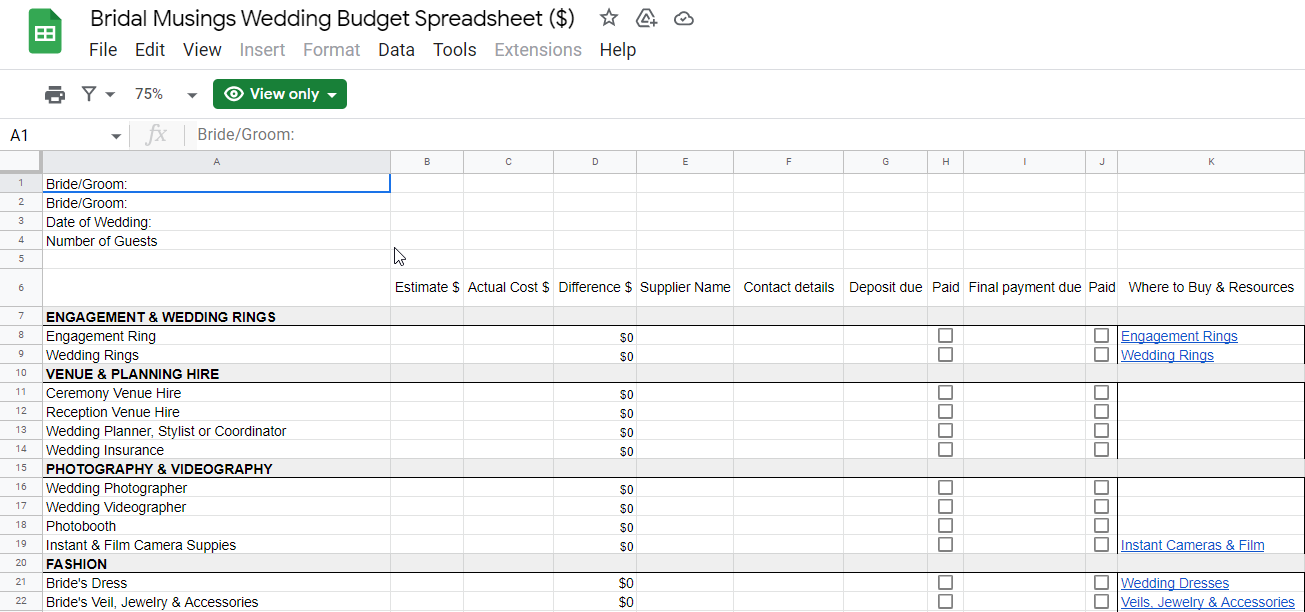This template needs to be opened via google sheet by default, if you need to use this template via Microsoft Excel spreadsheet, then you can download this wedding budget template spreadsheet for free by clicking on the Dropbox address here.

This template contains a variety of wedding essentials or some wedding services that you may need to purchase before and after the Wedding Day, and provides a record of the estimated and actual cost of these wedding essentials, and also automatically calculates the difference between the before and after pricing, so that you can easily calculate the amount over or under the budgeted price.

The template also provides a Where to Buy & Resources column to record the address of the online store where the current wedding item or service was purchased, allowing you to easily access the corresponding website to get instructions or customer reviews for the item or get some support from the online store’s customer service.

This template contains 3 simple Google sheets or Microsoft Excel formulas to automatically calculate the estimated cost, actual cost, over or savings of all wedding items or services purchased.

The three formulas are as follows:

```=SUM(B7:B119)

=SUM(C7:C119)

=B120-C120```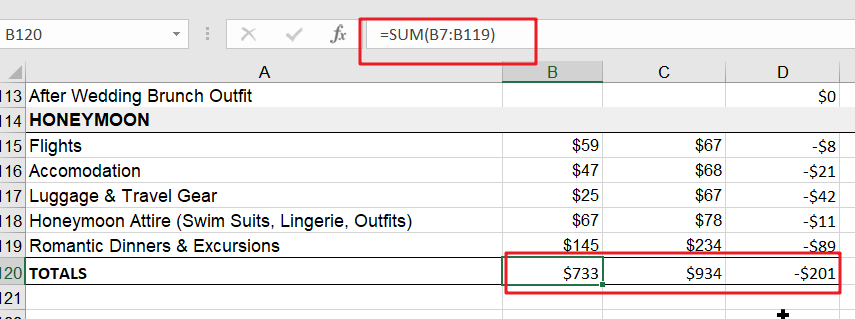The first formula calculates the total estimated amount for all items or services from B7 to B119 by using the SUM function, and the second formula automatically calculates the total actual amount spent for all items or services from C7 to C119 by using the SUM function. The third formula calculates overestimated or saved amount by subtracting the total estimated amount from the total spent amount.

As you can see from the image above, the current wedding cost is \$935 and the initial budget was \$733, so the actual cost was \$201 more than the initial budget.

If you have any other wedding cost items that you need to add to this template, then you can find the corresponding category and just insert a new line afterwards.

If you have any other personalization requirements, you can leave us a comment and we will assist you as well.

## 2. Wedding Budget Templates By APW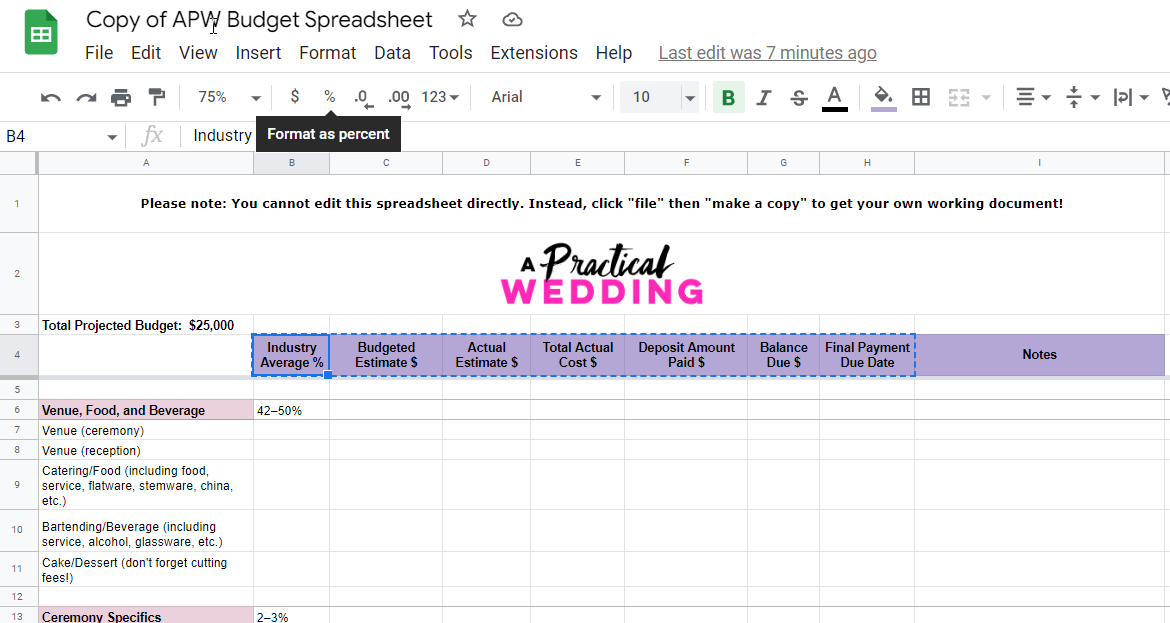This template needs to be opened through google sheets by default, if you need to use this template through Microsoft Excel Spreadsheet, then you need to download this template in xls format through google sheets and then use this free wedding budget template through Excel Spreadsheet.

This template is relatively simple and does not use any google sheets or Excel formulas, so it does not provide any complicated summation and statistical functions.

The following are the distinct features of this template:

• Provides some very useful statistics, such as industry averages for various wedding expense items, budgeted Estimate, Total Actual Cost, Deposit Amount Paid, Balance Due, Final Payment Due Date, etc. This allows you to easily track the current spending on wedding items.
• The header row of the template is set to freeze so that when you have a lot of wedding items or services, the header row will always be displayed and you can easily record the value of the corresponding column.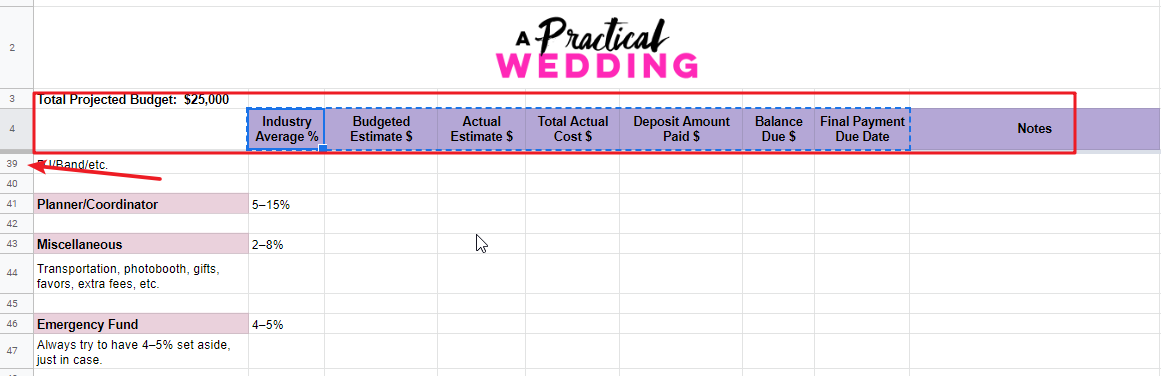This template can add some statistics through SUM function, for example, you can do summary statistics for all wedding items or services under a particular sub-category, such as total sub-category budget, total sub-category cost, total sub-category paid, etc.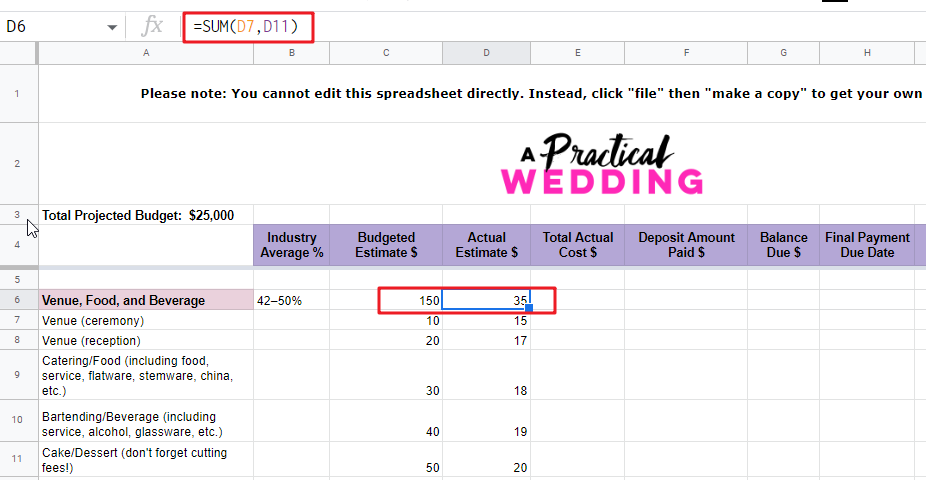Of course, you can also do summary statistics for all wedding items or services. If you have such customized needs, you can send us a message and we will assist you with these complex statistical functions.

## 3. Wedding Budget Templates By Microsoft Office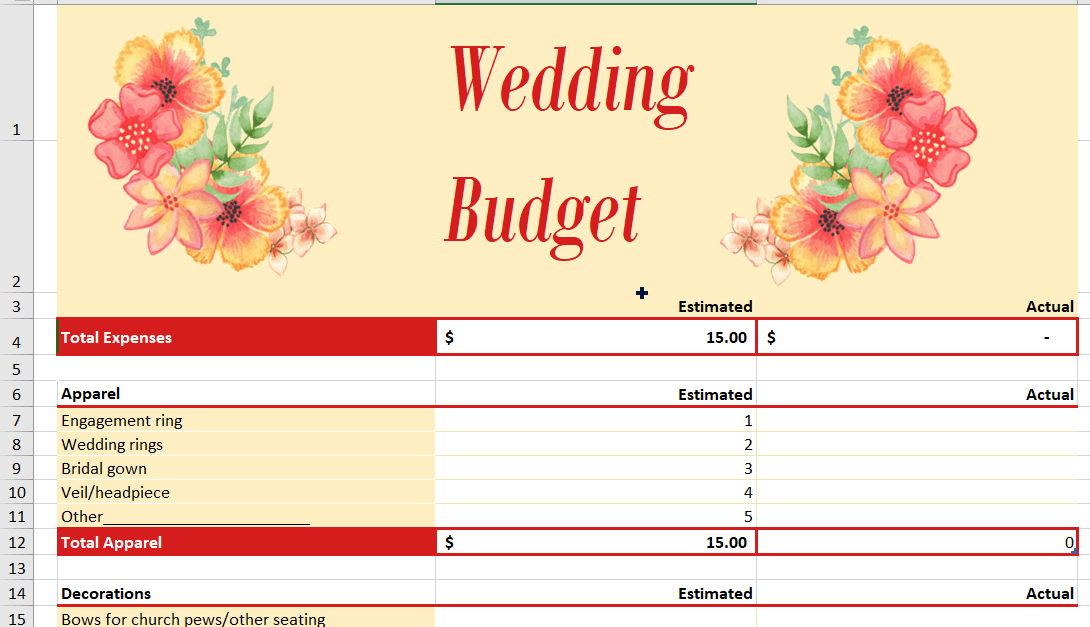This template is a free wedding budget spreadsheet provided by Microsoft, if you want to use this template in google sheets, then you can import it into google sheets.

The template provides a beautiful background image and the overall color of the spreadsheet is very nice; the template only provides the two most common statistical fields: Estimated and Actual, so the template is very simple and easy to use.

The top row of the template provides statistics for the total wedding budget and the actual cost, which are summarized for all wedding items and wedding services through the SUM function.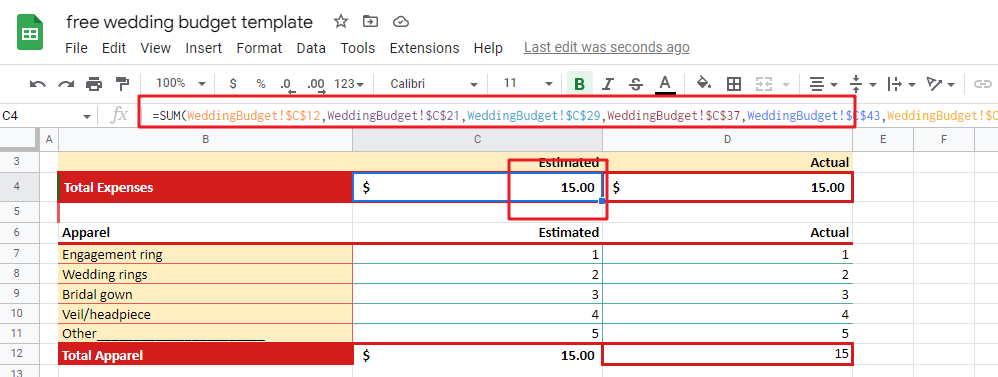This template provides total budget and total cost statistics for different wedding item categories by using the SUBTOTAL function. As the following figure shows.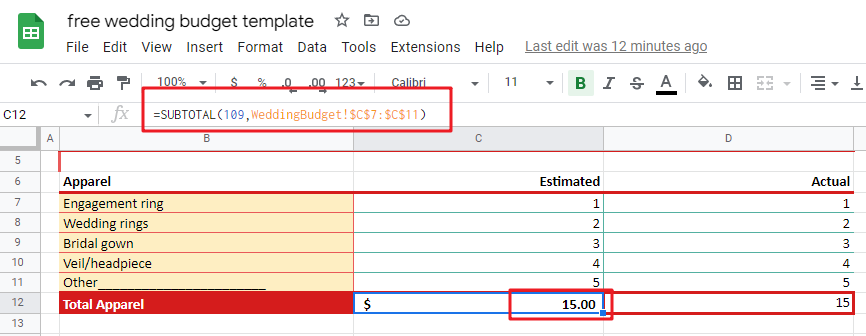## 4. Wedding Budget Templates By Vertex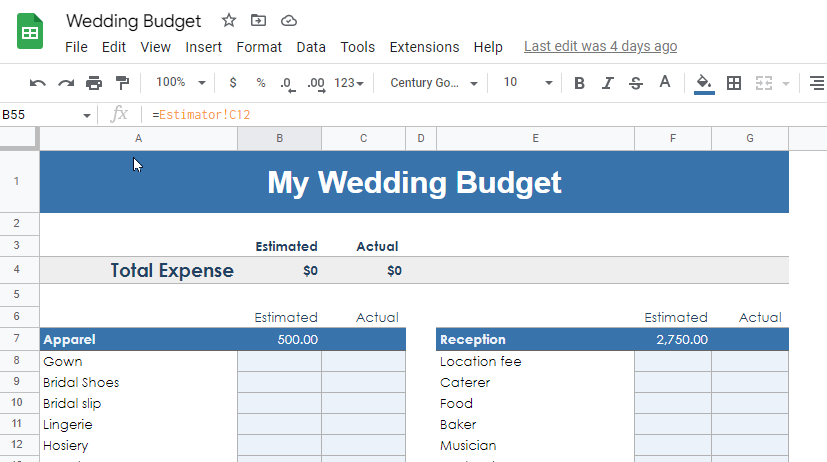This template needs to be opened through google sheets by default, if you need to use this template through Microsoft Excel Spreadsheet, then you need to download this template in xls format through google sheets and then use this free wedding budget template through Excel Spreadsheet.

This template is basically similar to the official Microsoft Office wedding budget template in Template 3, the only difference is that it puts the total budget statistics from the major categories of wedding items into a new Sheet. The advantage of this is that you can easily calculate the total budget automatically by adjusting the budget percentage for each major category. Please see the screenshot below：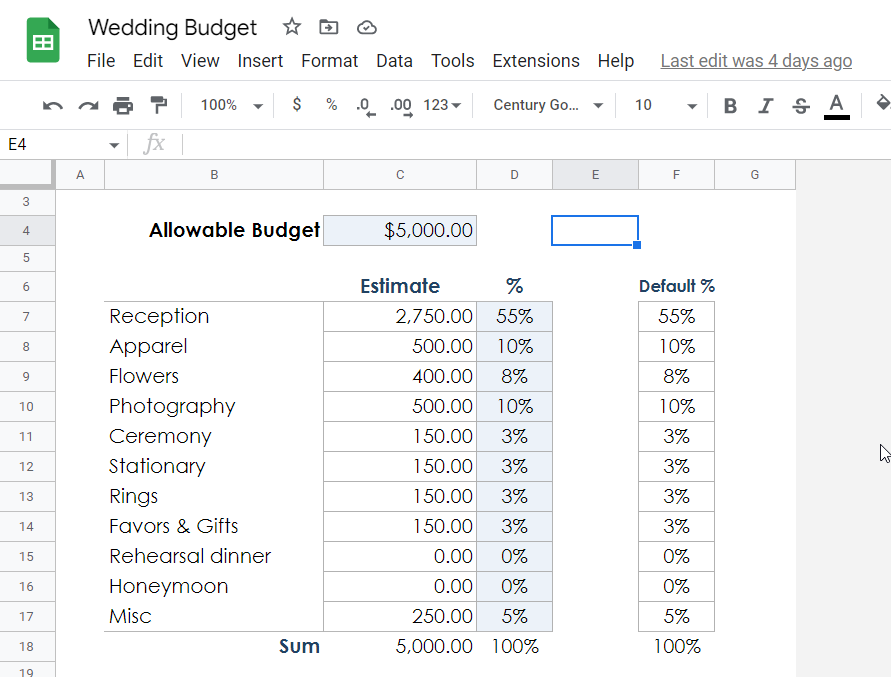This template is easy to use, the google sheets or Excel formulas in the template are very simple and just accumulate the cell values.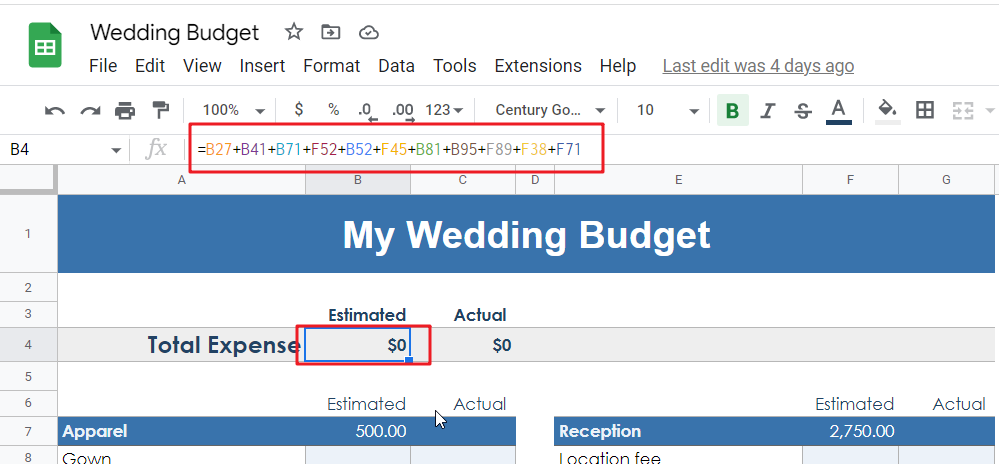If you have a more complicated customization requirement, you can send us a message and we can support you.

## 5. Wedding Budget Templates By SmartBride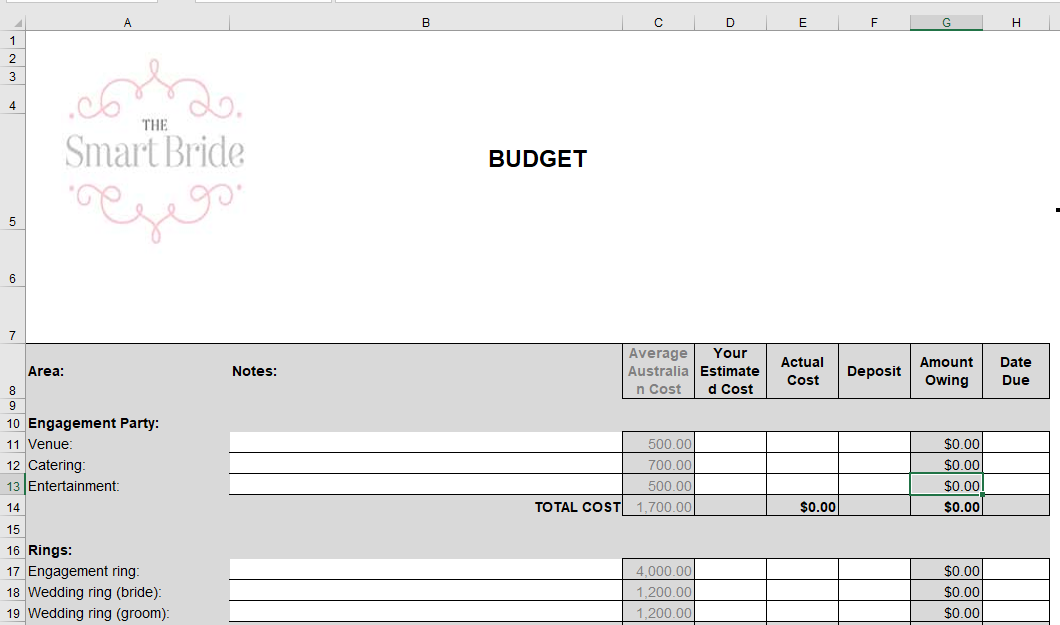This template is a free interactive wedding budget spreadsheet provided by After The Wedding, this template is also very easy to use. The template needs to be opened in Microsoft Excel by default, if you need to use this wedding budget template in Google Sheets, then you just need to import it.

The template is an overall wedding planning spreadsheet that includes a wedding calendar, wedding checklist, wedding supplier information, wedding guest template, wedding day schedule template and more.

The template also provides an average cost reference for wedding items, so you can easily compare it with the actual cost value.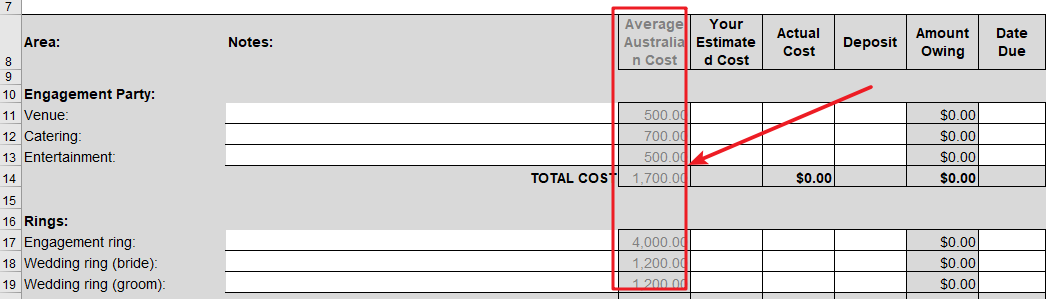## 6. Wedding Venue Tracking Spreadsheet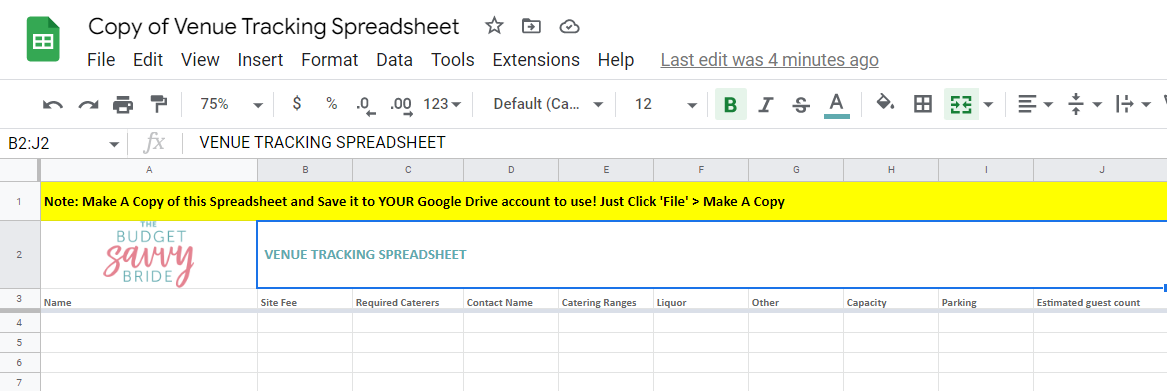This template needs to be opened through google sheets by default, if you need to use this template through Microsoft Excel Spreadsheet, then you need to download this template in xls format through google sheets and then you can open this free wedding budget template through Excel Spreadsheet.

The wedding reception will definitely take up most of the total wedding budget, and most wedding planners suggest that the wedding venue will take up 45% to 65% of the total budget. Therefore, if wedding venue rental costs are not reasonably controlled, it will definitely affect the cost of other wedding items or services.

This template provides a breakdown of costs for specific items at each venue, so you can easily track exactly what each venue offers and how much it will cost at the end of the wedding.

This template is a wedding venue information collection template and does not contain any google sheets or Excel formulas. If you need to personalize it, you can send us a message.

### Related Functions

• Excel SUM function
The Excel SUM function will adds all numbers in a range of cells and returns the sum of these values. You can add individual values, cell references or ranges in excel.The syntax of the SUM function is as below:= SUM(number1,[number2],…)…
The Google Sheets SUBTOTAL function returns the subtotal of the numbers in a list or database. The syntax of the SUBTOTAL function is as below:= SUBTOTAL (function_num, ref1, [ref2])….

## Calculate Cumulative Totals with Excel SUM Function

Today, through a simple example, we will show you how to use one of the most common-used Mathematical functions in excel, the SUM function, to add up the sum.

In our daily life, we keep an account of what we spend every day. For statistical purpose, we would like to know the total expenses in a period containing several consecutive days. The total expense is the expense of the day plus the expense of the previous days. As time passes, this value is accumulated. Since the total expense is dynamic and accumulated day by day, so to calculate the total spending of a period, we can use Excel SUM function with a mix reference.

Look at the example below. To calculate the total expense for a week, the starting day is Monday, and the ending day is Sunday. The user can check the total expense for any “consecutive days from Monday” of the week in the “Total” column.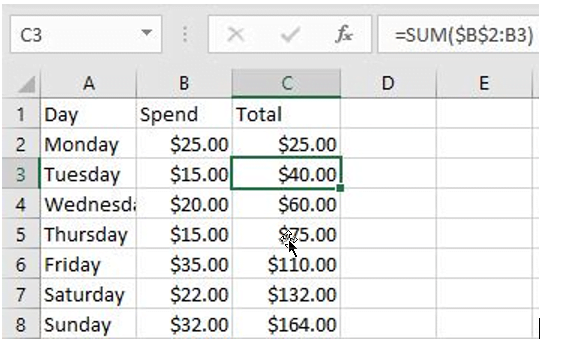In this example, the value in the “Total” column is the total expenses from Monday to that day.

## FORMULA

To sum a range with a locked starting cell, we can use Excel SUM function with a mixed reference. In this example, Monday through Sunday expenses are listed in the “Spend” column, the reference range is B2: B8. The starting day of a week is Monday, so the starting cell for calculating total expense is B2.

In this example, the formula is:

`=SUM(\$B\$2:B2)`

## EXPLANATION

In cell C2, the formula is =SUM(\$B\$2:B2).

Range \$B\$2:B2 only contains cell B2, so the value in B2 is returned in C2 directly.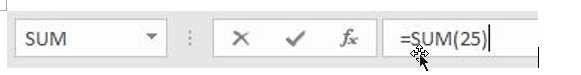Copy the formula down to C3 and the formula is updated to =SUM(\$B\$2:B3).

You can notice that after copying the formula by dragging the handle down, the starting cell is still B2. We lock this cell by adding \$ in front of the row and column indexes. Therefore, if the formula is copied down, this starting cell will not be changed.

The mixed reference is now correctly extended to \$B\$2:B3. So, the values in B2 and B3 are added up.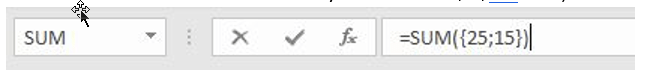As the formula was copied down, B2 was expanded to B3, B4 and up to B8 finally. the spending range was expanded to B8 at the end of the week.

• To calculate the total expense of Monday, Tuesday and Wednesday, the formula is =SUM(\$B\$2:B4).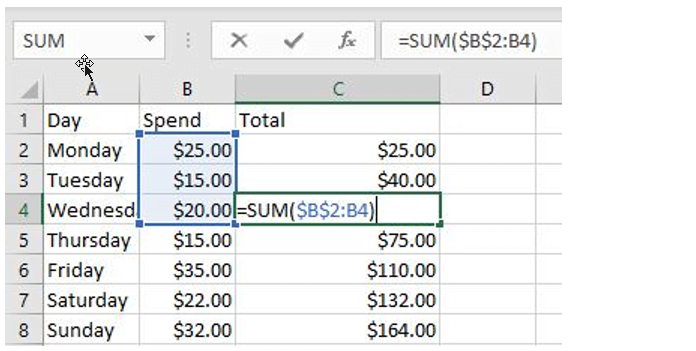• To calculate the total expense of a whole week, the formula is =SUM(\$B\$2:B8).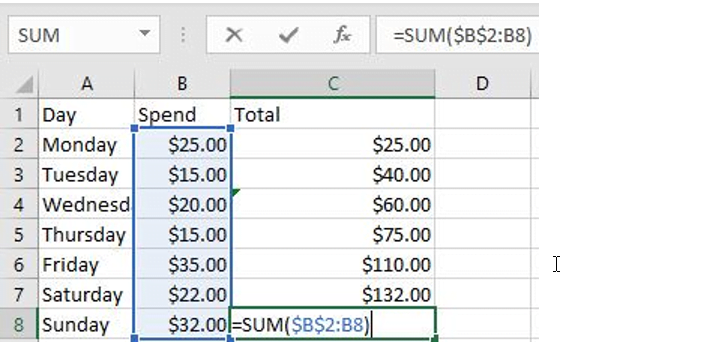### Related Functions

• Excel SUM function
The Excel SUM function will adds all numbers in a range of cells and returns the sum of these values. You can add individual values, cell references or ranges in excel.The syntax of the SUM function is as below:= SUM(number1,[number2],…)…